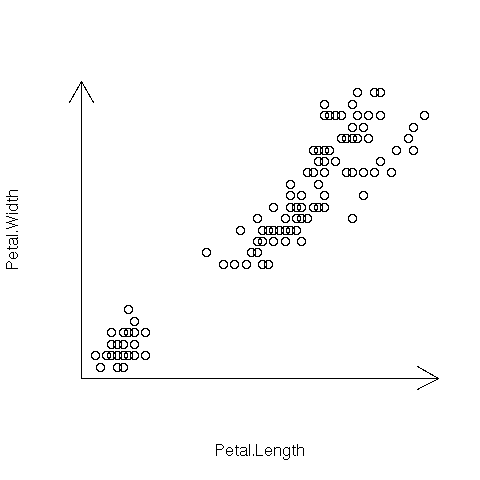DATA MINING
Desktop Survival Guide
by Graham Williams# Arrow Axes

The par function in R can be used to fine tune characteristics of a plot. Here we add arrows to the axes. For many more arrow options see the p.arrows function in the sfsmisc package.

In the example here, `par("usr")` is used to identify the bounds of the plot, and these are then employed to draw axes with arrows. The arrows function takes a starting point and an ending point for the line to be drawn. The `code=2` selects the type of arrow head to draw and the `xpd=TRUE` ensures the arrows are not cropped to the default size of the plot (the plot region).```attach(iris) plot(Petal.Length, Petal.Width, axes=FALSE) u <- par("usr") arrows(u, u, u, u, code=2, xpd=TRUE) arrows(u, u, u, u, code=2, xpd=TRUE) ```

http://rattle.togaware.com/code/rplot-iris-arrows.R